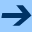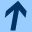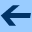Random number generation

# Random number generation

For games we often need to generate random numbers. We start by setting up a random number generator in a global variable:

  val random = java.util.Random()


Then, to generate a random integer in the range 0 to k-1, we call

  val random_number = random.nextInt(k)


You can also generate a random Double in the range $$0.0 \leq x < 1.0$$ using

  val x = random.nextDouble()Random number generation